If you have a related question, please click the "Ask a related question" button in the top right corner. The newly created question will be automatically linked to this question.

Part Number: TPS7A94

Hi, support team

My customer has the questions as follow:

In my design: input VIN = 2.5V, output VOUT = 1.8V, ICL = 80%

1. Which value should I choose for VFB_PG(typ) in Equation 3 when I calculate RFB_PG(TOP) and RFB_PG(BOTTOM) according to Equation 3?
0.2V? or 0.21V? or (VIN-VDO)~0.2V in Note1?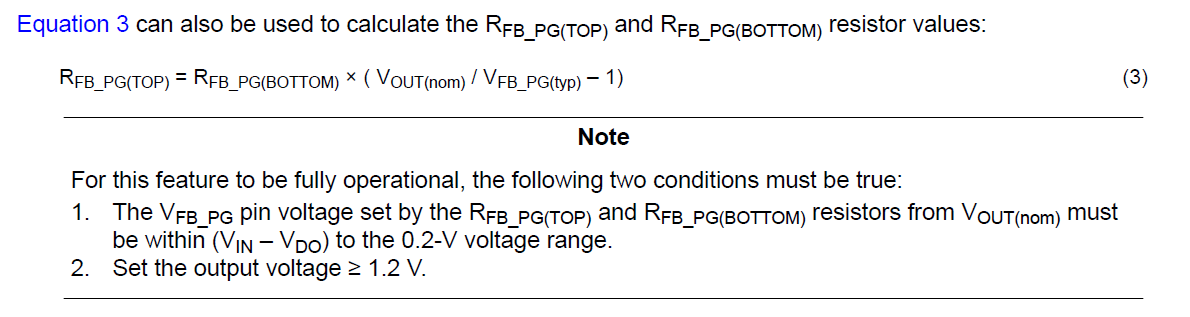2. How is the PG threshold parameter calculated in table 9-1?

Thanks so much.

Best regards,
Yuki

• There is one more question:

3. What is VEN_UV(HI) and VEN_UV(LOW) value?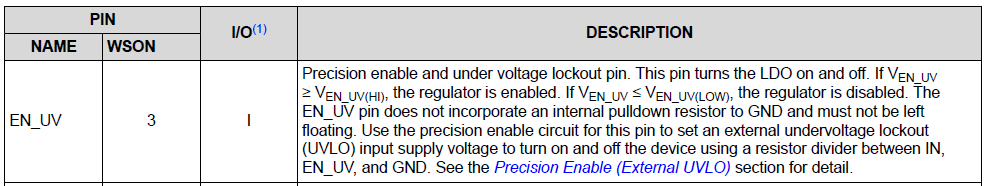Thanks so much.

• Hi Yuki-san,

1. The middle column is the typical value, so in this case you would use 0.2V. You can also use the min and max V_FB_PG specs to calculate the min and max PG threshold.
2. I'll use the VOUT(nom) = 5.1V last row case as an example. The V_FB_PG voltage when VOUT = VOUT(nom) is V_FB_PG = 5.1V * 100kΩ / (100kΩ + 2322kΩ) = 0.2105V. The PG trip point is 0.2V (typ), and so the PG threshold is then 0.2V / 0.2105V = .95 = 95% (typ) of the nominal voltage.
3. The specs are called V_IH(EN) and V_IL(EN) in the EC table. We will need to update the datasheet to keep a consistent naming convention. Thank you for bringing this to our attention.

Regards,

Nick

• Hi Nick,

In Figure 9-20, VOFF=3.75V, VON=4 V, Rtop=1.54 MΩ, Rbottom=100KΩ.
According to Equation 2, VEN_UV is not higher than V_IH(EN) ?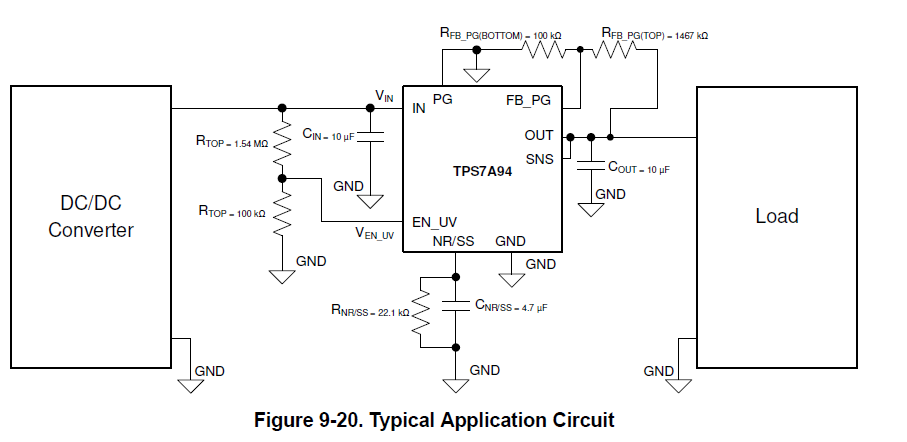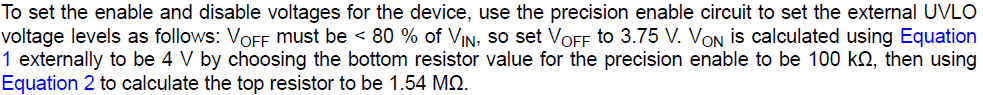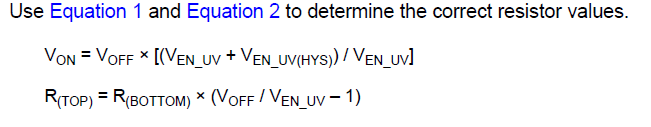Thanks so much.

Best regards,
Yuki

• Hi Yuki-san,

Yes that looks like another error. I calculated the R_TOP to be 212.5kΩ for this application. Does that sound right?

Regards,

Nick